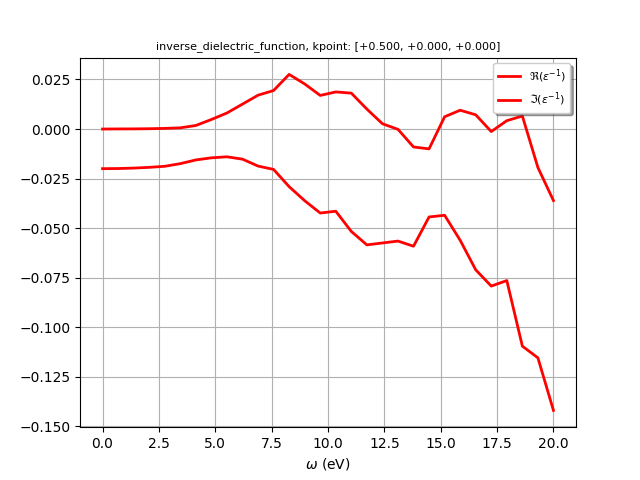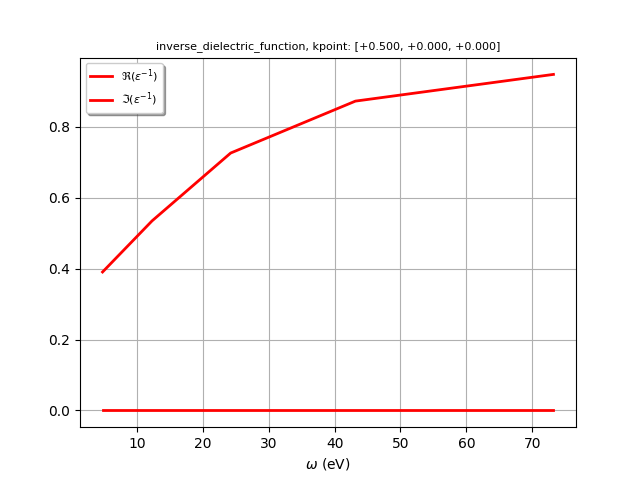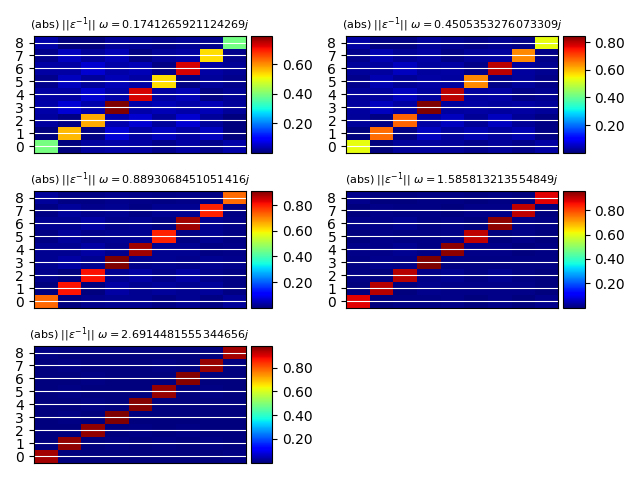# SCR matrix¶

This examples shows how to plot the matrix elements of the inverse dielectric function stored in the SCR file (optdriver 3) See also plot_scr.py for the optical spectrum.

•••Out:

======================== inverse_dielectric_function ========================
K-point: [+0.500, +0.000, +0.000], weight: 0.000
Number of G-vectors: 9
Total number of frequencies: 35 (real: 30, imaginary: 5)
Real frequencies up to 0.73 (eV)
Imaginary frequencies up to 2.69 (eV)
/Users/gmatteo/git_repos/pymatgen/pymatgen/util/plotting.py:550: UserWarning: Matplotlib is currently using agg, which is a non-GUI backend, so cannot show the figure.
plt.show()
/Users/gmatteo/git_repos/pymatgen/pymatgen/util/plotting.py:550: UserWarning: Matplotlib is currently using agg, which is a non-GUI backend, so cannot show the figure.
plt.show()


import abipy.data as abidata
from abipy.abilab import abiopen

with abiopen(abidata.ref_file("sio2_SCR.nc")) as ncfile:
#print(ncfile)
# The SCR file contains a structure and electron bands in the IBZ.
# We can thus use the ebands object to plot bands + DOS.
#ncfile.ebands.plot()

kpoint = [0.5, 0, 0]
print(em1)

gvec1 = [1, 0, 0]
gvec2 = [-1, 0, 0]

# Plot matrix element as function of frequency along the real axis
em1.plot_freq(gvec1=gvec1, gvec2=gvec2, waxis="real")

# Plot data along the imaginary axis
# Note that gvectors can also be specifined in terms of their index.
em1.plot_freq(gvec1=0, gvec2=0, waxis="imag")

# Plot e^{-1}_{G1, G2} along the imaginary axis.
em1.plot_gg(wpos="imag")


Total running time of the script: ( 0 minutes 0.775 seconds)

Gallery generated by Sphinx-Gallery